GeeksforGeeks App
Open AppBrowser
Continue

# Congruence of Triangles

Congruence of triangles is the property of two triangles in which all three corresponding sides and corresponding angles are equal. Two triangles are said to be congruent if and only if they can be overlapped with each other completely also, two triangles are said to be congruent if their corresponding sides and their corresponding angles are equal. There exist various conditions to prove congruency in triangles. In this article, all the necessary conditions to find congruency in triangles are discussed.

## Congruent Triangles

Congruence of any two figures is generally understood as two figures which can perfectly overlap each other. Congruent triangles are triangles that are perfect copies of one another. Various congruence rules are used to prove the congruency in two triangles. A triangle has six dimensions three sides and three angles any of the three dimensions given can be used to prove two triangles are congruent. More properties of triangles can be learned here.

Congruent triangles if arranged in proper orientation are mirror images of each other. Congruent objects are always superimposed images of one another. The corresponding angle and dimensions of congruent figures are the same. The symbol used to show congruency between two objects is “≅”. Two congruent triangles are given below.

To show congruency between them we write Δ ABC ≅ Δ PQR.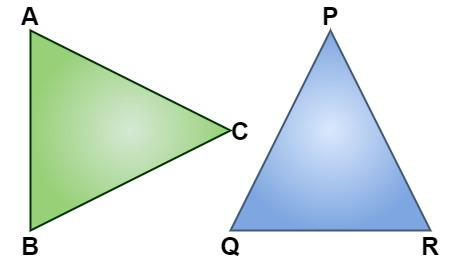## Congruent Triangles Postulates/Criteria

There are various methods to prove congruency among triangles. The methods which are used to prove congruency between two triangles are:

• SSS Criteria: Side-Side-Side
• SAS Criteria: Side-Angle-Side
• ASA Criteria: Angle-Side-Angle
• AAS Criteria: Angle-Angle-Side
• RHS Criteria: Right angle- Hypotenuse-Side

### SSS (Side, Side, Side)

In the postulate of SSS, if all three sides of a triangle are equal to the corresponding three sides of another triangle, then it satisfies the condition of SSS.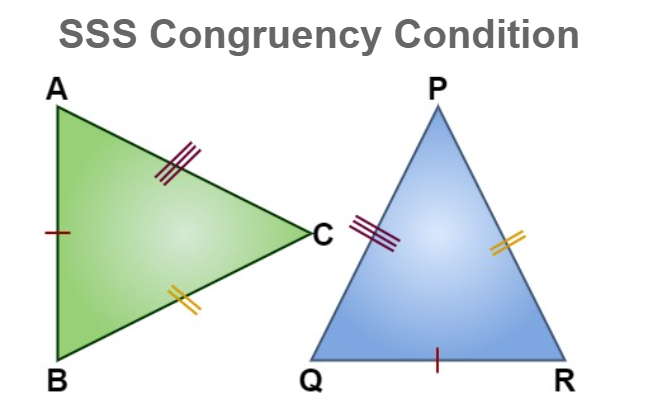In the above-given figure, we can see that,
AB = QR,
BC = RP, and
CA = PQ .
Hence, Δ ABC ≅ Δ QRP

### SAS (Side, Angle, Side)

In the postulate of SAS, in comparison among two triangles, if two sides and the angle included between two sides are equal. Then it satisfies the condition of SAS.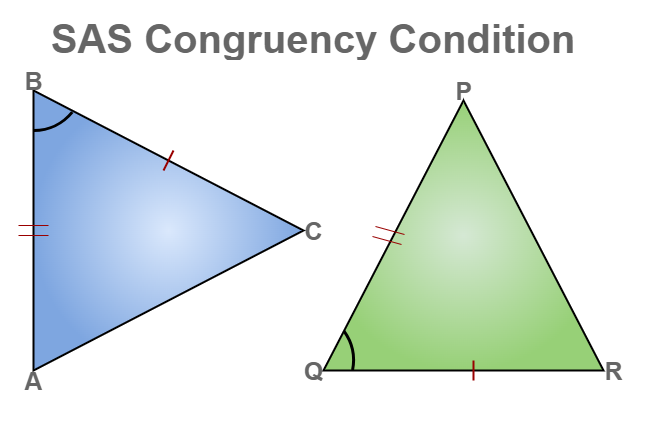In the above-given figure, we can see that,
CB = RQ,
BA = QP, and
∠B = ∠Q.
Hence, Δ CBA ≅ Δ RQP.

### ASA (Angle, Side, Angle)

Here, if we are having two triangles, where any two angles and sides included between the two angles of one triangle are equal to the angles and sides of the corresponding triangle. Then the two triangles will satisfy the ASA congruency.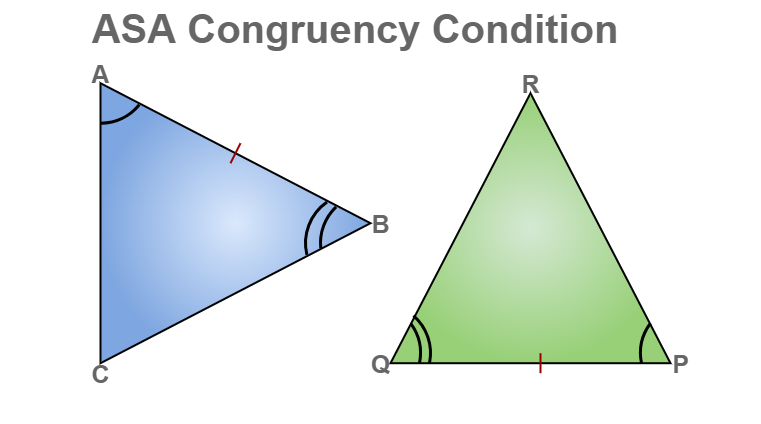In the above-given figure, we can see that,
AB = PQ,
∠A = ∠P, and
∠B = ∠Q.
Hence,  ΔACB ≅  ΔPQR.

### AAS (Angle, Angle, Side)

If any two angles of a triangle and non-included side are equal to the two angles and side of the corresponding triangle. Then it will satisfy the AAS congruency.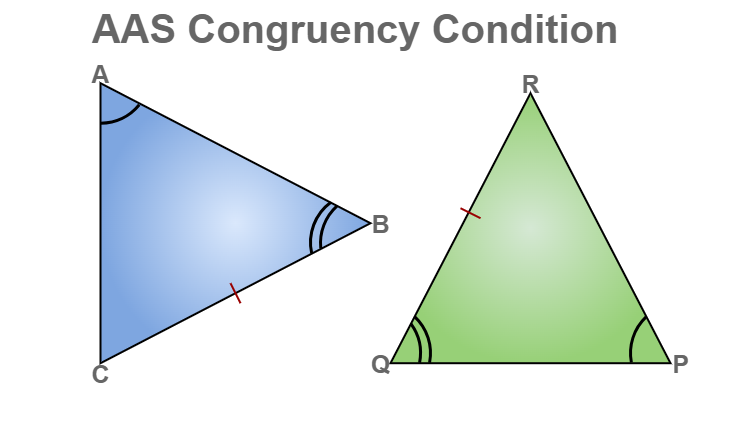From the given figure, we can say that,
BC = QR,
∠A = ∠P, and
∠B = ∠Q
Hence, ΔACB ≅  ΔDFE

### RHS (Right Angle- Hypotenuse- Side)

RHS criteria only get satisfied with a right-angled triangle. So, in RHS congruency, if only two sides i.e. hypotenuse and one side of a right-angled triangle are equal to the corresponding hypotenuse and side of another right-angled triangle. Then we can say that RHS is satisfied.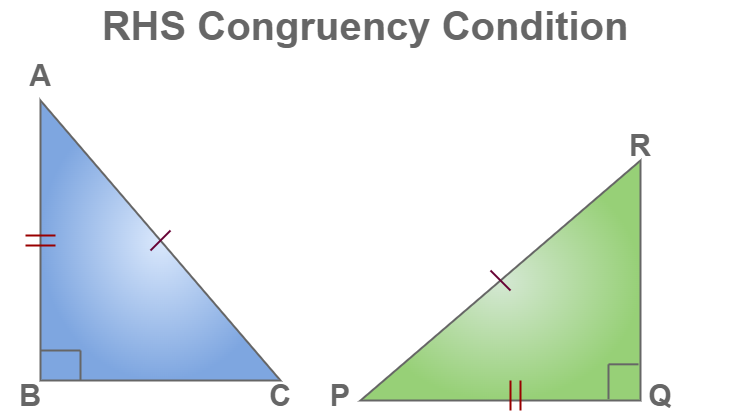From the above-given example of a right-angled triangle, we get
∠B = ∠Q (right angles)
CA = RP and
AB = PQ.
Hence, ΔACB ≅ ΔDFE.

## Properties of Congruent Triangles

Various properties of congruent triangles are

• Congruent Triangles are mirror images of each other.
• Congruent Triangles perfectly overlap each other if arranged in the proper orientation.
• Corresponding parts of Congruent Triangles are equal.
• Congruent Triangles have the same area.

## Solved Examples on Congruent Triangles

Example 1: Check whether the given triangles are congruent or not also, write the congruency criteria for congruency in triangles.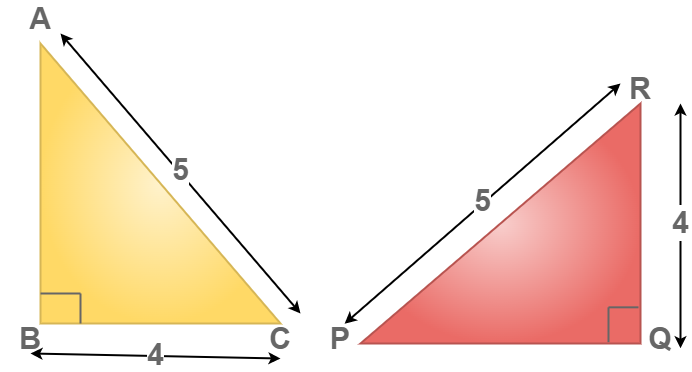Solution:

To identify the congruency in the above triangle.

BC = QR = 4 units (given)

CA = RP = 5 units (given)

∠ABC = ∠PQR = 90°

Now, we can conclude that Δ ABC ≅ Δ PQR by the RHS congruency condition.

Example 2: Check whether the given triangles are congruent or not also, write the congruency criteria for congruency in triangles.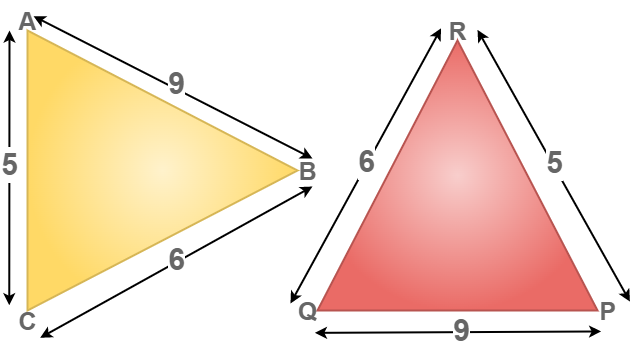Solution:

To identify the congruency in the above triangle.

BC = QR = 6 units (given)

CA = RP = 5 units (given)

AB = PQ = 9 units (given)

Now, we can conclude that Δ ABC ≅ Δ PQR by the SSS congruency condition.

Example 3: Check whether the given triangles are congruent or not also, write the congruency criteria for congruency in triangles.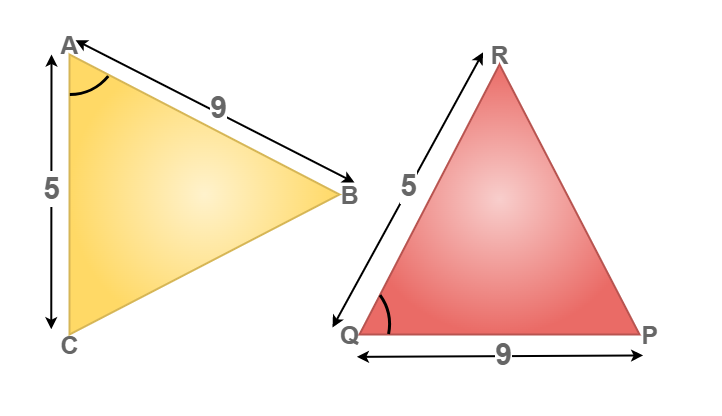Solution:

To identify the congruency in the above triangle.

AC = QR = 5 units (given)

∠BAC = ∠PQR (given)

BA = PQ = 9 units (given)

Now, we can conclude that Δ BAC ≅ Δ PQR by the SAS congruency condition.

Example 4: Check whether the given triangles are congruent or not also, write the congruency criteria for congruency in triangles.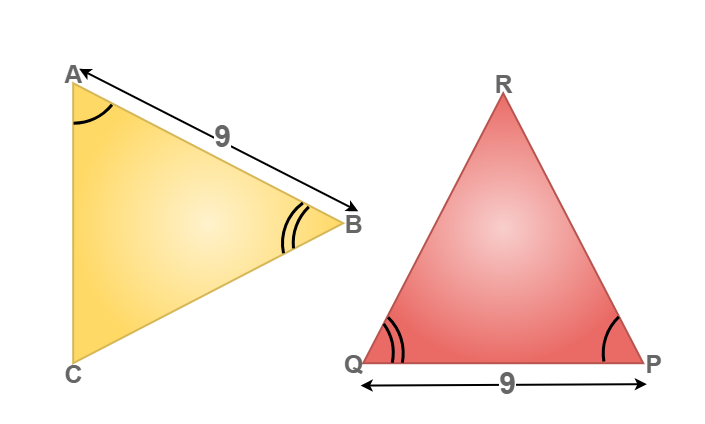Solution:

To identify the congruency in the above triangle.

∠BAC = ∠PQR (given)

BA = PQ = 9 units (given)

∠CAB = ∠QPR (given)

Now, we can conclude that Δ BAC ≅ Δ PQR by the ASA congruency condition.

Example 5: Check whether the given triangles are congruent or not also, write the congruency criteria for congruency in triangles.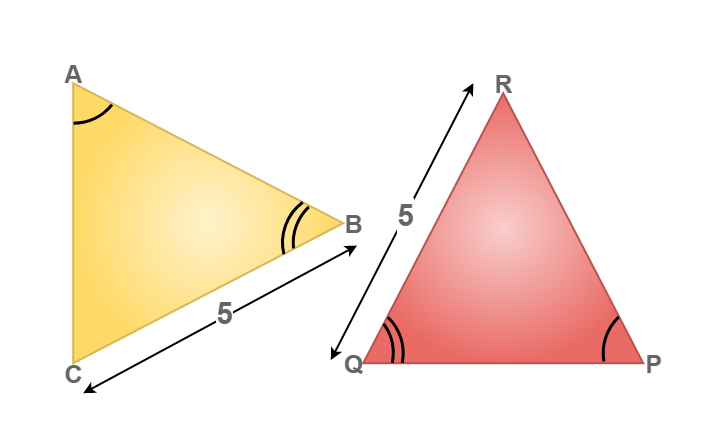Solution:

To identify the congruency in the above triangle.

BC = QR = 5 units (given)

∠ABC = ∠PQR (given)

∠BAC = ∠QPR (given)

Now, we can conclude that Δ ABC ≅ Δ PQR by the AAS congruency condition.

## FAQs on Congruent Triangles

Question 1: What are Congruent Triangles?

Congruent triangles are triangles that can overlap one another if arranged in a specific orientation. All three corresponding sides and corresponding angles in a congruent triangle are equal.

Question 2: How many criteria for congruency of the triangle?

The five main criteria for congruency of triangles are

SSS Rule: Side-Side-Side
SAS Rule: Side-Angle-Side
ASA Rule: Angle-Side-Angle
AAS Rule: Angle-Angle-Side
RHS Rule: Right angle- Hypotenuse-Side

Question 3: Write about the SSS congruency of triangles.

If all the three corresponding sides of two corresponding triangles are equal then the triangles are said to be congruent by SSS congruency condition.

Question 4: Is the AAA criterion used for proving congruency in triangles

No, AAA criterion cant be used for proving congruency in triangles. AAA criterion can be used for proving Similarities between two triangles.

Question 5: Write the Full Form of CPCT.

CPCT is an abbreviation for Corresponding Parts of Congruent Triangles. CPCT says if two or more triangles are congruent to each other, then the corresponding angles and the corresponding sides of the triangles are also congruent to each other.

Question 6: Write any two criteria for congruence of a triangle.

The two criteria of congruence of a triangle are:

• SSS congruency criteria
• SAS congruency criteria

Question 7: All similar triangles are congruent true or false.